Courses

# RD Sharma Solutions - Ex-15.2, (Part - 2), Properties Of Triangles, Class 7, Math Class 7 Notes | EduRev

## RD Sharma Solutions for Class 7 Mathematics

Created by: Abhishek Kapoor

## Class 7 : RD Sharma Solutions - Ex-15.2, (Part - 2), Properties Of Triangles, Class 7, Math Class 7 Notes | EduRev

The document RD Sharma Solutions - Ex-15.2, (Part - 2), Properties Of Triangles, Class 7, Math Class 7 Notes | EduRev is a part of the Class 7 Course RD Sharma Solutions for Class 7 Mathematics.
All you need of Class 7 at this link: Class 7

Q15.Find x,y,z(whichever is required) from the figures given below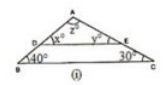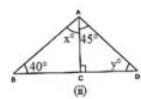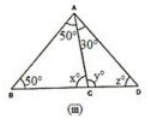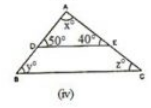Solution.

(i) In ΔABC and ΔADE we have :

x = 40

∠AED = ∠ACB (corresponding angles)

y = 30

We know that the sum of all the three angles of a triangle is equal to 180∘

x +y +z = 180 (Angles of A ADE)

Which means : 40 +30  + z = 180

z = 180−70

z = 110

Therefore, we can conclude that the three angles of the given triangle are 40, 30  and 110.

(ii) We can see that in ΔADC, ∠ADC  is equal to 90.

We also know that the sum of all the angles of a triangle is equal to 180.

Which means : 45+ 90 +y = 180 (Sum of the angles of ΔADC)

135  + y = 180

y = 180 – 135.

y = 45.

We can also say that in ΔABC,  ∠ABC+∠ACB+∠BAC  is equal to 180.

(Sum of the angles of A ABC)

40 + y + (x + 45) = 180

40 + 45 + x + 45 = 180                                                 (y = 45)

x = 180 –130

x = 50

Therefore, we can say that the required angles are 45 and 50.

(iii) We know that the sum of all the angles of a triangle is equal to 180.

Therefore, for ΔABD:

50 + x + 50  = 180

100  +x = 180

x = 180 –100

x = 80

For ΔABC:

∠ABC+∠ACB+∠BAC = 180 (Sum of the angles of ΔABC)

50 + z + (50 + 30) = 180

50 + z + 50 + 30 = 180

z = 180 – 130

z = 50

Using the same argument for ΔADC:

y +z +30 =180

y + 50∘ + 30 =180    (z = 50)

y = 180∘ – 80

y = 100

Therefore, we can conclude that the required angles are 80, 50 and 100.

(iv) In ΔABC and ΔADE we have :

y = 50

Also, ∠AED =∠ACB  (Corresponding angles)

z = 40

We know that the sum of all the three angles of a triangle is equal to 180.

Which means : x+50 +40 = 180  (Angles of ΔADE)

x = 180– 90

x = 90

Therefore, we can conclude that the required angles are 50, 40 and 90.

Q16. If one angle of a triangle is 60∘ and the other two angles are in the ratio 1 :2, find the angles

Solution.

We know that one of the angles of the given triangle is 60. (Given)

We also know that the other two angles of the triangle are in the ratio 1 : 2.

Let one of the other two angles be x.

Therefore, the second one will be 2x.

We know that the sum of all the three angles of a triangle is equal to 180.

60 +x + 2x = 180

3x =180– 60

3x = 120

x = 120/3

x = 40

2x = 2 x 40

2x = 80

Hence, we can conclude that the required angles are 40 and 80.

Q17. It one angle of a triangle is 100∘ and the other two angles are in the ratio 2 : 3. find the angles.

Solution.

We know that one of the angles of the given triangle is 100.

We also know that the other two angles are in the ratio 2 : 3.

Let one of the other two angles be 2x.

Therefore, the second angle will be 3x.

We know that the sum of all three angles of a triangle is 180.

100 + 2x + 3x = 180

5x = 180– 100

5x = 80

x = 80/5

2x = 2 x 16

2x = 32

3x = 3 x 16

3x = 48

Thus, the required angles are 32  and 48.

Q18. In ΔABC, if 3∠A = 4∠B = 6∠C, calculate the angles.

Solution.

We know that for the given triangle, 3∠A=6∠C

∠A = 2∠C—(i)

We also know that for the same triangle, 4∠B = 6∠C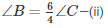We know that the sum of all three angles of a triangle is 180∘.

Therefore, we can say that:

∠A+∠B+∠C=180 (Angles of ΔABC)—(iii)

On putting the values of ∠Aand∠B in equation (iii), we get :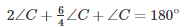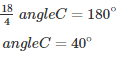From equation (i), we have:

angleA = 2∠C = 2×40

angleA = 80

From equation (ii), we have: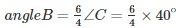angleB = 60

angleA = 80,  angleB = 60,  angleC = 40

Therefore, the three angles of the given triangle are 80, 60, and 40.

Q19. Is it possible to have a triangle, in which

(i) Two of the angles are right?

(ii) Two of the angles are obtuse?

(iii) Two of the angles are acute?

(iv) Each angle is less than 60?

(v) Each angle is greater than 60?

(vi) Each angle is equal to 60

Solution.

(i) No, because if there are two right angles in a triangle, then the third angle of the triangle must be zero, which is not possible.

(ii) No, because as we know that the sum of all three angles of a triangle is always 180. If there are two obtuse angles, then their sum will be more than 180, which is not possible in case of a triangle.

(iii) Yes, in right triangles and acute triangles, it is possible to have two acute angles.

(iv) No, because if each angle is less than 60, then the sum of all three angles will be less than 180, which is not possible in case of a triangle.

Proof:

Let the three angles of the triangle be ∠A, ∠B  and ∠C.

As per the given information,

∠A < 60  … (i)

∠B< 60   …(ii)

∠C< 60   … (iii)

On adding (i), (ii) and (iii), we get :

∠A + ∠B + ∠C < 60+ 60+ 60

∠A + ∠B + ∠C < 180

We can see that the sum of all three angles is less than 180, which is not possible for a triangle.

Hence, we can say that it is not possible for each angle of a triangle to be less than 60.

(v) No, because if each angle is greater than 60, then the sum of all three angles will be greater than 180, which is not possible.

Proof:

Let the three angles of the triangle be ∠A, ∠B  and ∠C. As per the given information,

∠A > 60  … (i)

∠B>60   …(ii)

∠C> 60   … (iii)

On adding (i), (ii) and (iii), we get:

∠A + ∠B + ∠C > 60+ 60+ 60

∠A + ∠B + ∠C > 180

We can see that the sum of all three angles of the given triangle are greater than 180, which is not possible for a triangle.

Hence, we can say that it is not possible for each angle of a triangle to be greater than 60.

(vi) Yes, if each angle of the triangle is equal to 60 , then the sum of all three angles will be 180 , which is possible in case of a triangle.

Proof:

Let the three angles of the triangle be ∠A, ∠B  and ∠C. As per the given information,

∠A = 60  … (i)

∠B = 60   …(ii)

∠C = 60   … (iii)

On adding (i), (ii) and (iii), we get:

∠A + ∠B + ∠C = 60+ 60+ 60

∠A + ∠B + ∠C = 180

We can see that the sum of all three angles of the given triangle is equal to 180, which is possible in case of a triangle. Hence, we can say that it is possible for each angle of a triangle to be equal to 60.

Q20. In ΔABC, ∠A = 100, AD bisects ∠A and AD perpendicular BC. Find ∠B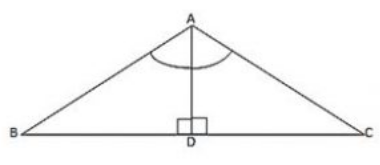Solution.

Consider ΔABD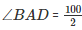(AD bisects ∠A)

We know that the sum of all three angles of a triangle is 180.

Thus,

Or,

∠ABD + 50 + 90 = 180

∠ABD = 180– 140

∠ABD = 40

Q21. In ΔABC,  ∠A = 50, ∠B=100  and bisector of ∠C  meets AB in D. Find the angles of the triangles ADC and BDC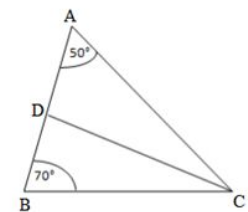Solution.

We know that the sum of all three angles of a triangle is equal to 180.

Therefore, for the given ΔABC, we can say that :

∠A + ∠B + ∠C = 180 (Sum of angles of ΔABC)

50∘ + 70∘ + ∠C  = 180

∠C = 180 –120

∠C = 60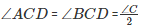(CD bisects ∠C  and meets AB in D.)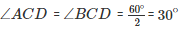Using the same logic for the given ΔACD, we can say that :

∠DAC  + ∠ACD  + ∠ADC  = 180

If we use the same logic for the given ΔBCD, we can say that

∠DBC   +∠BCD   +∠BDC   = 180

70 + 30 + ∠BDC   = 180

∠BDC   = 180 – 100

∠BDC   = 80

Thus,

For ΔADC: ∠A = 50, ∠D = 100   ∠C = 30

ΔBDC: ∠B = 70, ∠D = 80   ∠C = 30

Q22. In ΔABC, ∠A=60, ∠B=80,  and the bisectors of ∠B  and ∠C,  meet at O. Find

(i) ∠C

(ii) ∠BOC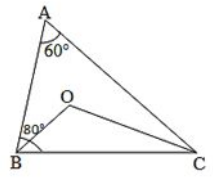Solution.

We know that the sum of all three angles of a triangle is 180.

Hence, for ΔABC, we can say that :

∠A + ∠B + ∠C = 180 (Sum of angles of ΔABC)

60+ 80∘ + ∠C = 180.

∠C = 180 – 140

∠C = 140.

For ΔOBC,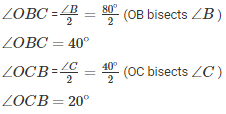If we apply the above logic to this triangle, we can say that :

∠OCB + ∠OBC + ∠BOC = 180 (Sum of angles of ΔOBC)

20+ 40  +∠BOC  = 180

∠BOC = 180 – 60

∠BOC = 120

Q23. The bisectors of the acute angles of a right triangle meet at O. Find the angle at O between the two bisectors.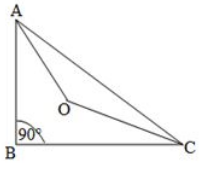Solution.

We know that the sum of all three angles of a triangle is 180∘.

Hence, for ΔABC , we can say that :

∠A + ∠B + ∠C = 180

∠A  + 90  + ∠C  = 180

∠A  + ∠C  = 180 – 90

∠A  + ∠C = 90

For ΔOAC   :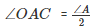(OA bisects LA)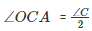(OC bisects LC)

On applying the above logic to ΔOAC, we get :

∠AOC+ ∠OAC+∠OCA   = 180    (Sum of angles of ΔAOC)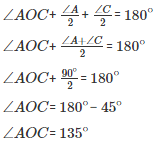Q24. In ΔABC, ∠A = 50 and BC is produced to a point D. The bisectors of ∠ABC  and ∠ACD meet at E. Find ∠E  .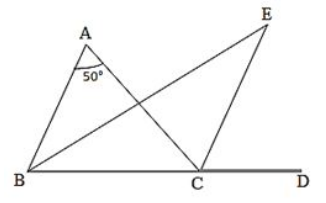Solution.

In the given triangle,

∠ACD = ∠A + ∠B. (Exterior angle is equal to the sum of two opposite interior angles.)

We know that the sum of all three angles of a triangle is 180.

Therefore, for the given triangle, we can say that :

∠ABC+ ∠BCA + ∠CAB = 180 (Sum of all angles of ΔABC)

∠A + ∠B + ∠BCA = 180

∠BCA = 180°- (∠A + ∠B)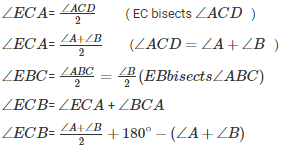If we use the same logic for ΔEBC , we can say that :

∠EBC+ ∠ECB + ∠BEC = 180 (Sum of all angles of ΔEBC)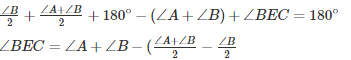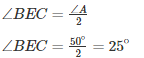Q25. In ΔABC, ∠B=60, ∠C=40, AL  perpendicular BC and AD bisects ∠A  such that L and D lie on side BC. Find ∠LAD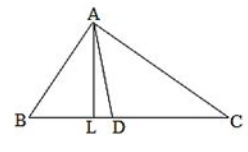Solution.

We know that the sum of all angles of a triangle is 180

Therefore, for ΔABC, we can say that :

∠A + ∠B + ∠C = 180

Or,

∠A + 60 + 40∘ = 180

∠A = 80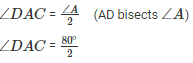If we use the above logic on ΔADC, we can say that :

∠ADC + ∠DCA + ∠DAC = 180 (Sum of all the angles of ΔADC)

∠ADC + 40 + 40 = 180

∠ADC = ∠ALD + ∠LAD(Exterior angle is equal to the sum of two Interior opposite angles.)

100 = 90+ ∠LAD       (AL perpendicular to BC)

Q26. Line segments AB and CD intersect at O such that AC perpendicular DB. It ∠CAB = 35  and ∠CDB = 55 . Find ∠BOD.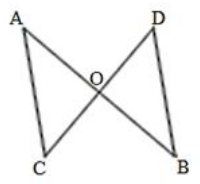Solution.

We know that AC parallel to BD and AB cuts AC and BD at A and B, respectively.

∠CAB = ∠DBA (Alternate interior angles)

∠DBA = 35

We also know that the sum of all three angles of a triangle is 180∘.

Hence, for ΔOBD, we can say that :

∠DBO + ∠ODB+ ∠BOD = 180

35 + 55 + ∠BOD = 180 (∠DBO =∠DBA and ∠ODB =∠CDB)

∠BOD =180 − 90

∠BOD = 90

Q27. In Fig. 22, ΔABC   is right angled at A, Q and R are points on line BC and P is a point such that QP perpendicular to AC and RP perpendicular to AB. Find ∠P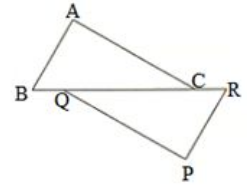Solution.

In the given triangle, AC parallel to QP and BR cuts AC and QP at C and Q, respectively.

∠QCA = ∠CQP (Alternate interior angles)

Because RP parallel to AB and BR cuts AB and RP at B and R, respectively,

∠ABC = ∠PRQ (alternate interior angles).

We know that the sum of all three angles of a triangle is 180.

Hence, for ΔABC, we can say that :

∠ABC + ∠ACB + ∠BAC = 180

∠ABC + ∠ACB + 90 = 180 (Right angled at A)

∠ABC + ∠ACB = 90

Using the same logic for ΔPQR, we can say that :

∠PQR + ∠PRQ + ∠QPR = 180

∠ABC + ∠ACB + ∠QPR =180∘ (∠ABC = ∠PRQ and ∠QCA =∠CQP)

Or,

90 + ∠QPR = 180 (∠ABC+ ∠ACB = 90)

∠QPR = 90

97 docs

,

,

,

,

,

,

,

,

,

,

,

,

,

,

,

,

,

,

,

,

,

,

,

,

,

,

,

,

,

,

,

,

,

;